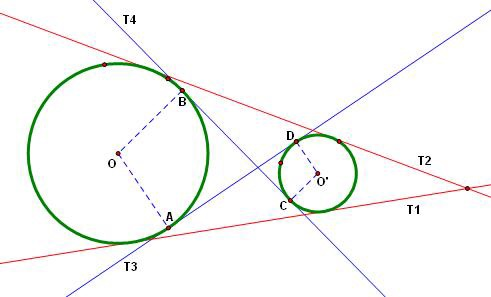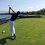# Common tangent rulesProblem 29 on the JEE mains inspired this note:

Things to know

•Pythagorean theorem

•Graphical transformations (only vertical and horizontal shifts)

This note will describe a quick and easy way to find the number of common tangents to two circles.

Given the following equations for the circles:

$(y-a)^2+(x-b)^2=r^2$

$y^2+x^2=s^2$

find the number of common tangents these circles will have.

You may wonder, "this isn't generalized, you assume one is at the origin". Let me explain.

What I did was move both circles $p$ units along the x-axis and $q$ units along the y-axis to make one at the origin. The number of tangents to the circles won't change if we perform a transformation other than a distortion.

Now for the tangent rules: how many common tangents will they have if...

I) $a^2+b^2=(r+s)^2$ then there are 3 common tangents.

II) $a^2+b^2>(r+s)^2$ then there are 4 common tangents.

Now for case 3

III) $a^2+b^2<(r+s)^2$ then we have three cases

NOTE: you must check this first, don't immediately check the rules below (Quick rule, if r=s in this case, then there are 2 tangents).

III-1) $a^2+b^2=(r-s)^2$ then there is 1 common tangent.

III-2) $a^2+b^2>(r-s)^2$ then there are 2 common tangents.

III-3) $a^2+b^2<(r-s)^2$ then there are no common tangents.Note by Trevor Arashiro
6 years, 2 months ago

This discussion board is a place to discuss our Daily Challenges and the math and science related to those challenges. Explanations are more than just a solution — they should explain the steps and thinking strategies that you used to obtain the solution. Comments should further the discussion of math and science.

When posting on Brilliant:

• Use the emojis to react to an explanation, whether you're congratulating a job well done , or just really confused .
• Ask specific questions about the challenge or the steps in somebody's explanation. Well-posed questions can add a lot to the discussion, but posting "I don't understand!" doesn't help anyone.
• Try to contribute something new to the discussion, whether it is an extension, generalization or other idea related to the challenge.
• Stay on topic — we're all here to learn more about math and science, not to hear about your favorite get-rich-quick scheme or current world events.

MarkdownAppears as
*italics* or _italics_ italics
**bold** or __bold__ bold
- bulleted- list
• bulleted
• list
1. numbered2. list
1. numbered
2. list
Note: you must add a full line of space before and after lists for them to show up correctly
paragraph 1paragraph 2

paragraph 1

paragraph 2

[example link](https://brilliant.org)example link
> This is a quote
This is a quote
    # I indented these lines
# 4 spaces, and now they show
# up as a code block.

print "hello world"
# I indented these lines
# 4 spaces, and now they show
# up as a code block.

print "hello world"
MathAppears as
Remember to wrap math in $$ ... $$ or $ ... $ to ensure proper formatting.
2 \times 3 $2 \times 3$
2^{34} $2^{34}$
a_{i-1} $a_{i-1}$
\frac{2}{3} $\frac{2}{3}$
\sqrt{2} $\sqrt{2}$
\sum_{i=1}^3 $\sum_{i=1}^3$
\sin \theta $\sin \theta$
\boxed{123} $\boxed{123}$

## Comments

Sort by:

Top Newest

Can you tell me such way in finding common tangents to a circle &a ellipse ?

- 6 years, 2 months ago

Log in to reply

×

Problem Loading...

Note Loading...

Set Loading...